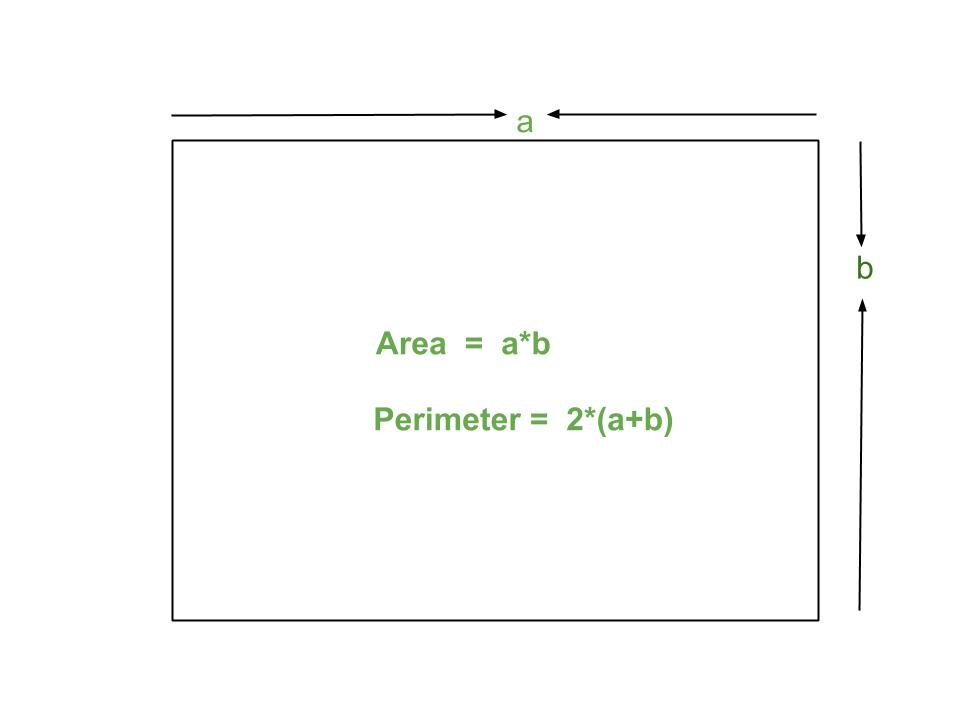Open In App

# Program for Area And Perimeter Of Rectangle

A rectangle is a flat figure in a plane. It has four sides and four equal angles of 90 degree each. In rectangle all four sides are not of equal length like square, sides opposite to each other have equal length. Both diagonals of the rectangle have equal length.Examples:

```Input : 4 5
Output : Area = 20
Perimeter = 18

Input : 2 3
Output : Area = 6
Perimeter = 10```

Formulae :

```Area of rectangle : a*b
Perimeter of rectangle: 2*(a + b) ```

## C++

 `// CPP program to find area``// and perimeter of rectangle``#include``using` `namespace` `std;`` ` `// Utility function``int` `areaRectangle(``int` `a, ``int` `b)``{``   ``int` `area = a * b;``   ``return` `area;``}`` ` `int` `perimeterRectangle(``int` `a, ``int` `b)``{``   ``int` `perimeter = 2*(a + b);``   ``return` `perimeter;``}`` ` `// Driver program``int` `main()``{``  ``int` `a = 5;``  ``int` `b = 6;``  ``cout << ``"Area = "` `<< areaRectangle(a, b) << endl;``  ``cout << ``"Perimeter = "` `<< perimeterRectangle(a, b);``  ``return` `0;``} `

## Java

 `// Java program to find area``// and perimeter of rectangle``import` `java.io.*;`` ` `class` `Geometry {`` ` `    ``// Utility function``    ``static` `int` `areaRectangle(``int` `a, ``int` `b)``    ``{``       ``int` `area = a * b;``       ``return` `area;``    ``}`` ` `    ``static` `int` `perimeterRectangle(``int` `a, ``int` `b)``    ``{``       ``int` `perimeter = ``2``*(a + b);``       ``return` `perimeter;``    ``}``     ` `    ``// Driver Function``    ``public` `static` `void` `main (String[] args) {`` ` `        ``int` `a = ``5``;``        ``int` `b = ``6``;``        ``System.out.println(``"Area = "``+ areaRectangle(a, b));``        ``System.out.println(``"Perimeter = "``+ perimeterRectangle(a, b));`` ` `    ``}``}`` ` `// This code is contributed by Chinmoy Lenka`

## Python3

 `# Python3 code to find area``# and perimeter of rectangle`` ` `# Utility function``def` `areaRectangle(a, b):``    ``return` `(a ``*` `b)`` ` `def` `perimeterRectangle(a, b):``    ``return` `(``2` `*` `(a ``+` `b))`` ` `# Driver function``a ``=` `5``;``b ``=` `6``;``print` `(``"Area = "``, areaRectangle(a, b))``print` `(``"Perimeter = "``, perimeterRectangle(a, b))`` ` `# This code is contributed by 'saloni1297'.`

## C#

 `// C# program to find area``// and perimeter of rectangle``using` `System;`` ` `class` `GFG {`` ` `    ``// Utility function``    ``static` `int` `areaRectangle(``int` `a, ``int` `b)``    ``{``        ``int` `area = a * b;``        ``return` `area;``    ``}`` ` `    ``static` `int` `perimeterRectangle(``int` `a, ``int` `b)``    ``{``        ``int` `perimeter = 2 * (a + b);``        ``return` `perimeter;``    ``}`` ` `    ``// Driver Function``    ``public` `static` `void` `Main()``    ``{`` ` `        ``int` `a = 5;``        ``int` `b = 6;``         ` `        ``Console.WriteLine(``"Area = "``                      ``+ areaRectangle(a, b));``        ``Console.WriteLine(``"Perimeter = "` `                 ``+ perimeterRectangle(a, b));``    ``}``}`` ` `// This code is contributed by vt_m.`

## PHP

 ``

## Javascript

 ``

Output :

```Area = 30
Perimeter = 22```

Time complexity : O(1)
Auxiliary Space : O(1)

This article is contributed by Saloni Gupta. If you like GeeksforGeeks and would like to contribute, you can also write an article using write.geeksforgeeks.org or mail your article to review-team@geeksforgeeks.org. See your article appearing on the GeeksforGeeks main page and help other Geeks.